1
In cart:
1 item\$2.50
Search and Filter Items
Search Products
OR Browse Departments

Filter Results

# Hot Dogs & Sausage

Product Size Price Quantity

CFGFL
16 oz
\$6.49

CFGFL
16 oz
\$7.99

## Bilinski Apple Chardonnay Chicken Sausage

GF
12 oz
\$6.99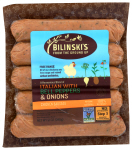## Bilinski Italian Pepper and Onion Chicken Sausage

GF
12 oz
\$6.99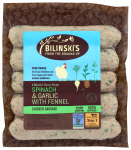## Bilinski Spinach, Garlic and Fennel Chicken Sausage

GF
12 oz
\$6.99GFOG
12 oz
\$8.99

CFL
Per Pound
\$11.99
lb

## Eichten’s Bison Sticks

CFL
Each (price approx.)
\$8.58You are ordering units, but this item is sold by the pound. The price per pound is \$25.99 and the average weight for one item is 0.33 lbs., therefore, the approximate price for one item is \$8.58. Please order the number of items you would like to receive. Your final price will be calculated based on the total weight of the actual items fulfilled.

## Eichten’s Bison Summer Sausage

CFL
Per Pound
\$17.99
lb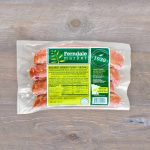CFL
12 oz
\$9.99

CFL
12 oz
\$9.99

CFL
12 oz
\$7.99

## Gerhard’s Smoked Brats

CFL
12 oz
\$7.99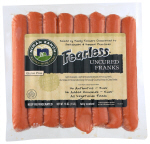16 oz
\$9.99

## Olli Gluten Free Chorizo Salami

GF
6 oz
\$12.99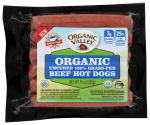CFLOG
10 oz
\$8.99

## Seward Co-op Andouille Pack Sausage

CFL
Each (price approx.)
\$15.98You are ordering units, but this item is sold by the pound. The price per pound is \$9.99 and the average weight for one item is 1.60 lbs., therefore, the approximate price for one item is \$15.98. Please order the number of items you would like to receive. Your final price will be calculated based on the total weight of the actual items fulfilled.

## Seward Co-op Andouille Sausage

CFL
Each (price approx.)
\$2.97You are ordering units, but this item is sold by the pound. The price per pound is \$8.99 and the average weight for one item is 0.33 lbs., therefore, the approximate price for one item is \$2.97. Please order the number of items you would like to receive. Your final price will be calculated based on the total weight of the actual items fulfilled.

## Seward Co-op Bratwurst

CFL
Each (price approx.)
\$2.80You are ordering units, but this item is sold by the pound. The price per pound is \$8.49 and the average weight for one item is 0.33 lbs., therefore, the approximate price for one item is \$2.80. Please order the number of items you would like to receive. Your final price will be calculated based on the total weight of the actual items fulfilled.

## Seward Co-op Bratwurst Pack

CFL
Each (price approx.)
\$15.18You are ordering units, but this item is sold by the pound. The price per pound is \$9.49 and the average weight for one item is 1.60 lbs., therefore, the approximate price for one item is \$15.18. Please order the number of items you would like to receive. Your final price will be calculated based on the total weight of the actual items fulfilled.

## Seward Co-op Breakfast Sausage

CFL
Each (price approx.)
\$3.30You are ordering units, but this item is sold by the pound. The price per pound is \$9.99 and the average weight for one item is 0.33 lbs., therefore, the approximate price for one item is \$3.30. Please order the number of items you would like to receive. Your final price will be calculated based on the total weight of the actual items fulfilled.

CFL
Per Pound
\$8.99
lb

CFL
Per Pound
\$7.49
lb

## Seward Co-op Calabrese Italian Sausage

CFL
Each (price approx.)
\$3.63You are ordering units, but this item is sold by the pound. The price per pound is \$10.99 and the average weight for one item is 0.33 lbs., therefore, the approximate price for one item is \$3.63. Please order the number of items you would like to receive. Your final price will be calculated based on the total weight of the actual items fulfilled.## Seward Co-op Chicken Hot Dog Pack

CFL
Each (price approx.)
\$9.99You are ordering units, but this item is sold by the pound. The price per pound is \$9.99 and the average weight for one item is 1.00 lbs., therefore, the approximate price for one item is \$9.99. Please order the number of items you would like to receive. Your final price will be calculated based on the total weight of the actual items fulfilled.

## Seward Co-op Chicken Italian Sausage

CFL
Each (price approx.)
\$3.30You are ordering units, but this item is sold by the pound. The price per pound is \$9.99 and the average weight for one item is 0.33 lbs., therefore, the approximate price for one item is \$3.30. Please order the number of items you would like to receive. Your final price will be calculated based on the total weight of the actual items fulfilled.

## Seward Co-op Chicken Provencal Sausage

CFL
Each (price approx.)
\$2.97You are ordering units, but this item is sold by the pound. The price per pound is \$8.99 and the average weight for one item is 0.33 lbs., therefore, the approximate price for one item is \$2.97. Please order the number of items you would like to receive. Your final price will be calculated based on the total weight of the actual items fulfilled.

## Seward Co-op Chorizo

CFL
Each (price approx.)
\$3.30You are ordering units, but this item is sold by the pound. The price per pound is \$9.99 and the average weight for one item is 0.33 lbs., therefore, the approximate price for one item is \$3.30. Please order the number of items you would like to receive. Your final price will be calculated based on the total weight of the actual items fulfilled.

## Seward Co-op Chorizo Chub

CFL
Each (price approx.)
\$8.99You are ordering units, but this item is sold by the pound. The price per pound is \$8.99 and the average weight for one item is 1.00 lbs., therefore, the approximate price for one item is \$8.99. Please order the number of items you would like to receive. Your final price will be calculated based on the total weight of the actual items fulfilled.

## Seward Co-op Currywurst

CFL
Each (price approx.)
\$2.97You are ordering units, but this item is sold by the pound. The price per pound is \$8.99 and the average weight for one item is 0.33 lbs., therefore, the approximate price for one item is \$2.97. Please order the number of items you would like to receive. Your final price will be calculated based on the total weight of the actual items fulfilled.

## Seward Co-op Fig and Shallot Sausage

CFL
Each (price approx.)
\$3.30You are ordering units, but this item is sold by the pound. The price per pound is \$9.99 and the average weight for one item is 0.33 lbs., therefore, the approximate price for one item is \$3.30. Please order the number of items you would like to receive. Your final price will be calculated based on the total weight of the actual items fulfilled.

## Seward Co-op Franklin Frank Pack

CFL
Each (price approx.)
\$9.99You are ordering units, but this item is sold by the pound. The price per pound is \$9.99 and the average weight for one item is 1.00 lbs., therefore, the approximate price for one item is \$9.99. Please order the number of items you would like to receive. Your final price will be calculated based on the total weight of the actual items fulfilled.

## Seward Co-op Italian Meatballs Pack Sausage

CFL
Each (price approx.)
\$10.99You are ordering units, but this item is sold by the pound. The price per pound is \$9.99 and the average weight for one item is 1.10 lbs., therefore, the approximate price for one item is \$10.99. Please order the number of items you would like to receive. Your final price will be calculated based on the total weight of the actual items fulfilled.

## Seward Co-op Maple Breakfast Sausage

CFL
Each (price approx.)
\$2.97You are ordering units, but this item is sold by the pound. The price per pound is \$8.99 and the average weight for one item is 0.33 lbs., therefore, the approximate price for one item is \$2.97. Please order the number of items you would like to receive. Your final price will be calculated based on the total weight of the actual items fulfilled.

## Seward Co-op Mild Italian Sausage Chub

CFL
Each (price approx.)
\$7.49You are ordering units, but this item is sold by the pound. The price per pound is \$7.49 and the average weight for one item is 1.00 lbs., therefore, the approximate price for one item is \$7.49. Please order the number of items you would like to receive. Your final price will be calculated based on the total weight of the actual items fulfilled.

## Seward Co-op Polish Smoked Sausage

CFL
Each (price approx.)
\$2.97You are ordering units, but this item is sold by the pound. The price per pound is \$8.99 and the average weight for one item is 0.33 lbs., therefore, the approximate price for one item is \$2.97. Please order the number of items you would like to receive. Your final price will be calculated based on the total weight of the actual items fulfilled.

## Seward Co-op Smoked Brat Pack

CFL
Each (price approx.)
\$15.98You are ordering units, but this item is sold by the pound. The price per pound is \$9.99 and the average weight for one item is 1.60 lbs., therefore, the approximate price for one item is \$15.98. Please order the number of items you would like to receive. Your final price will be calculated based on the total weight of the actual items fulfilled.

## Seward Co-op Smoked Cheddar Brat

CFL
Each (price approx.)
\$2.50You are ordering units, but this item is sold by the pound. The price per pound is \$9.99 and the average weight for one item is 0.25 lbs., therefore, the approximate price for one item is \$2.50. Please order the number of items you would like to receive. Your final price will be calculated based on the total weight of the actual items fulfilled. 1 in cart Go to Cart

## Seward Co-op Smoked Cheddar Pack Bratwurst

CFL
Each (price approx.)
\$16.78You are ordering units, but this item is sold by the pound. The price per pound is \$10.49 and the average weight for one item is 1.60 lbs., therefore, the approximate price for one item is \$16.78. Please order the number of items you would like to receive. Your final price will be calculated based on the total weight of the actual items fulfilled.

## Seward Co-op Spring Chicken Pack Sausage

CFL
Each (price approx.)
\$15.83You are ordering units, but this item is sold by the pound. The price per pound is \$10.99 and the average weight for one item is 1.44 lbs., therefore, the approximate price for one item is \$15.83. Please order the number of items you would like to receive. Your final price will be calculated based on the total weight of the actual items fulfilled.

## Seward Co-op Spring Chicken Sausage

CFL
Each (price approx.)
\$3.30You are ordering units, but this item is sold by the pound. The price per pound is \$9.99 and the average weight for one item is 0.33 lbs., therefore, the approximate price for one item is \$3.30. Please order the number of items you would like to receive. Your final price will be calculated based on the total weight of the actual items fulfilled.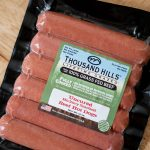CFGFL
10 oz
\$6.99

CFGFL
10 oz
\$8.99

CFL
2 oz
\$5.99